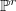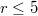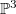﻿

### Postulation of general unions of lines and multiplicity two points in$\mathbb {P}^r$,$r \leq 5$

#### Abstract

We determine the Hilbert function of general unions in$\mathbb {P}^r$ , r = 4; 5, of prescribed numbers of lines and fat 2-points. We only have partial results in$\mathbb {P}^3$ (also if we add a few general reducible conics).

DOI Code: 10.1285/i15900932v35n1p23

Keywords: postulation; Hilbert function; lines; zero-dimensional schemes; fat points

Full Text: PDF